## Tuesday, 31 March 2009

### Testing Understanding of Slope

As part of our professional growth and school improvement process, several members of my department and I have formed a focus group to study formative assessment; testing which focuses on testing to make instructional decisions rather than for grading. One of our goals is to try and develop a deeper understanding of how to write better questions in order to measure student learning.

Recently I imposed on several members of my staff to give a brief assessment to their students that included two different forms of a question on slope. The questions, shown below, were of the lines y=3/4 x -3 and y=3/4 x - 2. I assumed their would be a slight difference in the number of correct responses since one had integer intercepts on both the x and y axis, and one did not.What I didn't expect was the degree of difference. The students ranged from Algebra I students who have only recently been introduce to slope, through geometry students who had reviewed the topic twice this year according to their teacher, my own sections of Alg II students whom I assumed were much stronger on the topic than my results made me know, and a section of statistics students; many of whom are taking an additional pre-calc or calculus class. In all there were 47 students who took each form. Of the 47 who had the question with integers on both intercepts, a total of 26, or 55%, got the correct answer. Of the 47 who had the line translated one unit up the y-axis, only 10, or 21% gave the correct response.

Four more of the students who had the "easier" question had the expected types of mistakes; they either gave the reciprocal of the correct response, or the negative of it. I was not prepared for how strongly the students were bound to the x and y intercepts. Thirteen of the students using the second form had given slopes of .8 or 2/2.5 using the values they interpreted as the intercepts. Of the 22 students in Alg II who took the questions, 8 of 11 gave the correct answer for the "easier" problem, but only three of them were correct (that is, they answered 3/4) on the second form. Five more of them, however, gave the answer of 2/2.5 or .8 to the second form. There is no question in my mind that they understood the idea of slope. I have no quarrel with the people who would give full credit to the answer of .8 or 4/5. There is however, I think, some difference between the student who focuses solely on the intercepts and the ones who see the whole line, coordinate plane relationship. I think a question which differentiates between them tells me something about how they see and treat slope.

I guess I am more concerned than ever about learning how to write good assessment items that will help me, and my students, understand what they know, and don't know, about the mathematical ideas we are covering.

## Saturday, 28 March 2009

### Isoperimetric problems and scale

We had just finished that old classic about finding the minimum surface area of a can (closed cylinder) if the volume was fixed. I had given them a volume of 128 Pi cubic cm so that when they were done the radius and height would come out in integer units, four and eight cm respectively. Many found the minimum surface area to be 96 Pi square units and the ones who finished quickly were helping the others find their way through the substitution of h=128/r2 into the formula for surface area so that they could graph the function, or perhaps work out intricate details of arithmetic, "but 256 divided by 4 is 64, not 84." Finally we were ready, I thought, for a little closure.

"So, what did you notice?" Many hands up.... I point, they answer.. "The minimum is 96." I hesitated, perhaps even made a slight face of despair. I had hoped they would notice more. They picked up on the "That's not what I'm looking for" face and someone quickly added, "96 cubic centimeters... no meters, no, wait, I mean square centimeters.." amidst some laughter on their part I tried to draw them deeper into the problem. "Well, yes, that is the minimum for THIS problem, but what else did you notice?"

With long troubled faces, they poured through their solutions, what was the old man after now... we got the answer... finally a brave soul offers a trial balloon..."The surface area is less than the volume?"; drawn out as a question.
We had recently talked about the isoperimetric problems, planer figures with the same number for the area and perimeter, and I had talked about, and solved the cube with the same number for its volume and surface area... (I have several blogs a while ago on this idea; here and here and a third one here) which I assume made him think to compare the results in this fashion.
"So... " I asked. They somehow took that as encouragement and someone conjectured that the very act of "minimizing" the surface area meant it would be smaller than the volume. That seemed easy enough to counter, but I still wanted them to notice one other thing, so I thought a minute about which way to adjust and gave a second version of the same problem..."Find the minimum surface area of a cylinder with a volume of 16 pi cubic cm."
They sped quickly through the now-familiar problem, and announced that the minimum area was 24 Pi cubic inches. I waited, eyebrows lifted. A moment later the quickest realizes.... audibly..."Damn," and then to the student on his left, "It's bigger... its more than the volume!".

"So what ELSE do you notice, what seems to be true in both cases?" I ask. They struggle, nothing seems obviously the same. Someone notices, and offers, almost apologetically, that both the radius and height in the second problem were 1/2 of original solution .

I was willing to drag this out to make them think, so I asked her if she thought the radius and height would continue to diminish by 1/2 in every problem I could think up. Finally she restated what she thought might be a truism..."The radius is half the height, is that always true?"

"Well, let's test it." Earlier in the year they would have groaned when their answers led to another problem, but by now they expected that every question led to another question. This time I picked an intermediate value, knowing I only had one more chance to offer integer values, I suggested they try 54 pi cubic cm. They sped through the problem and found that indeed the height was 6, twice the radius value of 3. They were so glad to feel like they were on the track of something they didn't even notice that this time the surface area also had a numeric value of 54 pi.

I had allowed myself to get hung up between two big ideas, now I had to kill one to chase the other, so I just summarized their radius is one-half height in a simple statement. "Ok, so what have we found? The can which most efficiently contains a given volume of substance will have a height that is 2r, or equal to its diameter. It is the same distance across as it is up and down." I have always intended, and never followed up on, to make a solid that meets this equality. Maybe now I will; because the next thing I told them was wrong. I said that it was close to a typical tuna fish can. (actually the ones in my cupboard are too short for the diameter. The closest I could find was a can of Eagle Brand that was about 3.25 inches high and about 3 inches in diameter.)

Finally I wanted to try once more to drive home a big idea about that classic relationship between length, area, and volume that seems forever to not seem obvious to even advanced students. I talk about how in one case the volume was more than the surface area, and in another the volume was less, and then, we stumble upon the "Goldilocks" size, where they both are "just right."

I spend some time drawing rectangles of every kind of size and showing that you can always make one with the same ratio of sides that has exactly the same number for area and perimeter, and the same thing is true for any two or three dimensional shape... and then I said it, "Every shape is isoperimetric under some measurement scale!" and the kid sitting in the front row, before he even thought, says "That can't be so?, there must be some shapes that won't work."

So I set them one more problem; "I have a solid shape so convoluted that it has no name It has a volume of 64, and a surface area of 512 units. Blowing it up and shrinking it is like changing from measuring in inches to feet or mm or something different so let's just change the numbers. If I make a scale model of it that is bigger or smaller, what happens to the volume and surface area?"

They want a formula... I refuse...I return to the original two cylinder problems and ask what is the relationship between the original radii... they finally recall that it was four cm in the first and 2 cm in the last, the ratio was 2:1. "What about the heights?" and again they come up with 2:1...

"Ok, what about the surface areas?" I get 96/24 a few times and someone realizes 4:1...
"The volumes????" and they start to recall... Oh yeah, 8:1 squares and cubes...

"So if the volume is 64 and the surface area is 512, what happens if I make the model half as big in any length?" They realize that heads them off the wrong way and begin to work on the solution..

Finally one comes to the board with a solution... The ratio of SA : Volume = 8:1. If we increase the size by a linear dilation of k, the new ratio will be k2 8 : k3 and we want that to equal one. so we just set k2 8 = k3 which means when k=8 the ratio and surface area are the same number. "And the original shape???" I ask... "Doesn't matter." he answers... and mercifully for all of us, the bell rings.

## Monday, 23 March 2009

### An Improved Solution to the Probability Problem

My last blog gave a labored explanation of why given R red balls and W white balls in a bowl, if they are removed one at a time randomly until one color has been totally removed, what is the probability that White will be exhausted first.

Joshua Zucker gave much more elegant expalantion for the reason the probability of white being exhausted first is red/(red + white)

His solution, in my words.. forget everything except the last ball in the bowl... whatever color it is is Not the color that was exhausted. So All we have to do to know the probability that White is exhausted, is find the probability that the last ball drawn if we emptied the bowl would be red. But if we look at all the possible orders of drawing all the balls, 4/7 of the first balls drawn are red, 4/7 of the second balls drawn are red..and the same continues all the way to the end; 4/7 of the final balls drawn will be red, meaning that in 4/7 of the trials, the white balls were exhausted first.

Now I'm thinking about the case of three colors, red, white and blue. I haven't started, so if you have an easy explanation, lay it on me.... otherwise, more later.

-------------- edited afterward...
ask and you shall receive... Joshua does what he does best, thinking and explaining his thoughts on the fly... see the comment and learn ....

## Sunday, 22 March 2009

### Probably an Interesting Problem

It was a simple probability problem, but it teased me with its surprising answer, and led me on … but let me start at the beginning…
A message on the AP discussion group from “Skerbie” (would I make this up?) asked…

If there are 4 red balls and 3 white balls in a container, and you remove them one at a time at random until one color is extinguished, what is the probability the remaining balls are red?

The simple answer, 4/7… soooo… would that always happen? And what is the math at work.

I quickly tried several examples where the number of white and red differed by one and they all seemed to preserve the ratio. At first I thought that it would only work with a difference of one, but with a wake up reminder from Joshua Zucker I corrected my arithmetic flaws and figured out that, Yes, the probability of the smaller color being extinguished first is the ratio of red/(red+white). SO… to show why….

One of the first things that I thought was interesting and (at least by me) unsuspected is that the probability of drawing WWW is the same as drawing WRWRW, or RRWWW, or RWWRW or any of the possible outcomes that ends with white being exhausted first. For the original seven problem case, the probability of drawing WWW is (3/7) (2/6)(1/5)= 1/35…The same probability applies to, for example, WRWRW which is (3/7)(4/6)(2/5)(3/4)(1/3) because the last two fractions simply cancel out the impact of the red and we get 1/35 again… I picked up pretty quickly that the 35 in the denominator was the combination of 7 things taken 3 at a time. I checked a few other starting quantities and this was true of the others as well, if you had n white balls, and n+d red balls, the probability of any of the paths ending with white being exhausted first led to a probability of 1 over 2n+d choose n.

As I looked at counting the possible outcomes ending in white being exhausted, I realized that :
There was only one way to draw WWW
There were three ways to draw them with one red in the mix if the red could not be at the end
RWWW; WRWW; WWRW
And there were six ways to draw them with two reds in the mix.
RRWWW, RWRWW; RWWRW; WRRWW; WRWRW; WWRRW
And there were ten ways to draw them with three reds in the mix… this was the sequence of triangular numbers, in particular I realized that if the last one HAD to be white, the sequence was counting 2 choose 2, and then 3 choose 1; 4 choose 2; 5 choose 3. But since all of those had the same probability of occurring, then the total probability was just the sum of those multiplied by 1/35. And the sum of the numbers down that diagonal of Pascal’s arithmetic triangle was 20, which is 6 choose 3….

I extended this to realize that as long as White had N balls, and Red had N+1, then there would be 2N choose N ways to order the outcomes, and each had a probability of 2N+1 choose N

In Pascal’s triangle, these were the successive numbers marked below:The first is for two reds and one white which leads to a probability of 2/3. The second is for three red and two white, and then for the four red, three white case. The 70/126 is the probability for starting with five red and four whites, and simplifies to the expected 5/9.

When we expand the values for 2N choose N and 2N+1 choose N,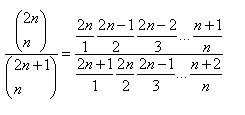we see why the result always leads to the result that the overall probably of white being exhausted first is Red/(Red + White) which, when there are N+1 Reds and N whites leads to (N+1) / (2N+1) .

A very similar approach shows that when the difference between the number of Reds and Whites in the bowl is two, then we just move over one column in the arithmetic triangle to get :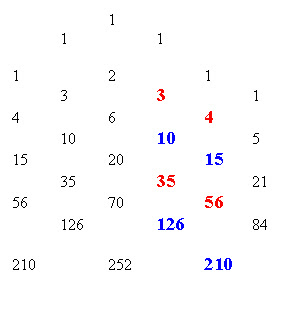Ok, Maybe I’m the only old guy on the block who didn’t expect that…. But I bet a fistful of nickels that any bright kid in Alg II could look at what just happened and know where to go to find the answer if Red was three more than White…

They have the arithmetic triangle dating back to at least the thirteenth century, so why am I just finding this stuff out….

## Friday, 20 March 2009

### Things I Learned at the Carnival

They just posted the newest "Math Teachers at Play", a math education carnival, this time hosted by Kate at f(t). There are lots of good reasons you should visit if you are a teacher of math at any level, a student, someone just interested in math, or just the curious kind of person who drops by my blog.
They started this time with a link to my post on centroids, "Just an Average Point"; which proves they must have wonderful stuff...
Ok, if that isn't enough to convince you, there are all kinds of cool things I picked up in just a few moments on a couple of the links... Did you know???
33 is the largest number that is not a sum of distinct triangular numbers. Which made me wonder, can you get them all with distinct triangular numbers using addition AND subtraction???? Haven't checked myself yet, but curious.

34 is the smallest number with the property that it and its neighbors have the same number of divisors. Hmmm? That's three in a row... can you find the smallest TWO consecutive numbers that have the same number of factors (ok,gotta be bigger than 2...

40 is the only number whose letters are in alphabetical order.... which has GOT to be a great trivia question. It also got me wondering...are there numbers in Spanish, Greek, Russian, Tagalog... ???? that are in alphabetical order, and is there a language that there is not ANY number that is spelled in alphabetical order? Ok, Help me out here readers!!!!!

And besides, there was this great "space invaders" cartoon... and if you are too young to know what space invaders was...well, heck, you are just TOO YOUNG.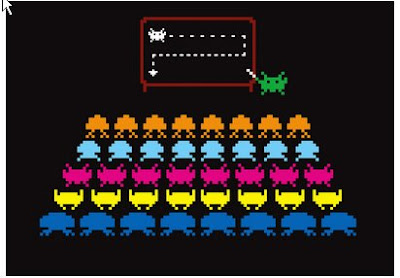## Wednesday, 18 March 2009

### Just an Average Point

Somewhere back in Alg I, we introduce the midpoint, and maybe some bright kid notices that the midpoint of (2, 7) and (6, 9) is just (4, 8); which is just the average of 2 and 6 followed by the average of 7 and 9; just average the x-values and average the y-values

Later in Geometry, we introduce midpoints all over the place, midpoints of a segment, as one end of the medians of a triangle, or as a point on the perpendicular bisector, but it is almost always in an abstract approach and they never actually have to ferret out the coordinates, so that seldom gets reinforced. I think that is a shame, because in the same course, we get another nice opportunity to introduce the 2 dimensional equivalent. One of the beautiful geometric ideas that still persists in a few geometry classes is that the three medians always intersect in a point. The point is called the centroid*, and sometimes the center of gravity. (Some teachers like to have students make triangles out of cardboard and after they find the centroid the students can cut it out and balance the cardboard triangle on a pencil tip.) If you use an interactive geometry software package, like sketchpad, you can actually construct the triangle and its medians, then grab a point and drag it around and see that...wow! no matter how you alter the shape of the triangle, the three medians still intersect in a single point. If you don't have the software to play with on your on, you can open a java applet I made here and click the mouse button on any of the triangle vertices and move them freely.

But almost no geometry class I know of goes on to show that the point is really sort of a "midpoint" of the triangle, and like the midpoint of a segment, it is just an "average" point. The x,y coordinates of the centroid are just the average of the x-coordinates of the three points followed by the average of the y-coordinates.
If, for example, we had triangle ABC with A=(1, 2); B= (5, 11) and C = (9, -1) then the medians will intersect at the point where x= (1+5+9)/3=5 and y= (2+11 - 1)/3 = 4; or more simply at the point (5,4)About this time of year as I am introducing my pre-calc class to the beauty of three dimensional analytic geometry, I dust off their memory of this little jewel from when I taught it in Alg II, and then we extend it to show it works with triangles in three-space. This requires them to a) Find the midpoints of the sides; b) write the equation the medians in the space triangle; c) find the intersection of two vector lines in space; (d) and then confirm that that point is also on the third line; and finally e) insure that it is indeed the average of the relative coordinates. I think it is a pretty good illustration of the power of the linear algebra they are learning.

As a reward, I give them a discovery lesson on tetrahedra... we talk for a minute about the difference between a two dimensional object in three space (A triangle with (x,y,z) coordinates for the points) and a three dimensional object (like a cube) . I bring out a large cardboard model of a tetrahedron (triangle based pyramid) and hold it in front of me..... and I begin to wonder aloud..." This is sort of like an extension of a triangle in three dimensions.... so what would be like the medians of a triangle in this object?"
Frequently the first guess is a line from a vertex to a point which is the "face-center" or centroid. I usually channel them (or prompt them if needed) toward this idea. "Wouldn't it be wonderful", I add, " if there was something like the centroid for the tetrahedron; where all those lines would intersect." By now they suspect that there is, and perhaps with some fear as they realize that they are about to be challenged to find one, or worse, prove it is always true.

With much the same vector approach as before, I pass out some cards with a set of four points in space (carefully chosen to make the work a little less messy than it might be), and they find centroids of the four faces, hopefully using the "average point" method. Then they must write vector equations of the four lines which I call "medial" lines that run from a vertex of the tetrahedron to the centroid of the opposite face. Finally I suggest that they solve the intersections of the four medials in sets of two, and behold that the two solutions are the same point; all four lines intersect in a single point.

Then I ask, "What is the quickest way to find the midpoint of a line segment?" "Averages", they shout.

"What is the quickest way to find the centroid of a triangle?" "Averages", some reply, but others already have anticipated the next question, and have started to calculate the average of the four x values.... could it be?

As a finale I point out that there is a pattern in the division of the segments in each of the cases..
The midpoint divides the line segment (a one space object) into a ratio of 1:1
The centroid divides the segment of a triangle (a two space object) into a ratio of 2:1
The center of gravity of a tetrahedron ( a three space object) divides the medial segments in a ratio of 3:1

Just another "average" day in mathematics class.

If you want a little more about centroids, see my web page article.

*There are three common "centers of gravity" that are studied in math, science and engineering. The most common in math is the center of masses located at the vertices of a polygon. This is more common because the other two cases can be reduced to a variation of this approach. It is this case of point masses at the vertices that I mean when I use centroid or center of gravity in this note, unless otherwise stated. A second approach is to treat the area of the polygon as if it were a sheet of uniform density. The third, and least common, approach is to represent the sides of the polygon as wire rods of uniform density.

Most students are first introduced to the terms above in reference to a point in a triangle. Since the center of masses at the vertices in a triangle give the same point as a uniform sheet, they are often confused about the various distinctions. The three centers of gravity are usually different points in other non-symmetric polygons. It is this point, the center of balance for the uniform sheet and also of point masses at the vertices, that is almost universally referenced as the centroid of a triangle.

## Tuesday, 17 March 2009

### The Pinhole Effect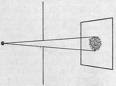David Craig, an excellent teacher with the DoDDS System in Germany wrote to tell me, "Pat, your “Tiny Circles” blog got me motivated to finally make this Sketchpad simulation that I’ve had in my head for awhile. "
The blog was about the way an image viewed through a pinhole becomes more clear (although less bright)
David included a link to a Geometers Sketchpad demo that he made to illustrate both of these effects to his students, and gave me permission to share. If you have the GSP software, you can download the demo here.
Thanks for sharing David.

## Sunday, 15 March 2009

### Catalan Asymptotic Function

I have written before about the Catalan Functions, named for Eugene Catalan of Belgium (1814-1894). I recently came across a note on the "God Plays Dice" blog that gave an asymptotic function for the Catalan Numbers (an Asymptotic function gives you a value it approaches as N gets larger and closer to infinity).. The value is given asThe nice part of this is that if n is pretty big, you can ignore all the stuff in parentheses. For example the 6th Catalan number is 132, and the value outside the parentheses is about 157.. ok, a little high, but as you work your way up, the error is smaller and smaller (at least percentage wise)... and always an upper bound (too much)....
There was also a cute joke of the kind that only a matematician could appreciate, "identify the sequence "una, dues, cinc, catorze, quaranta-dues, cent trenta-dues, quatre-cent vint-i-nou,...", which are the Catalan numbers 1, 2, 5, 14, 42, 132, 429... in the Catalan language."

Catalan Numbers first appeared in disguise in a problem Euler first proposed to Christian Goldbach in 1751. The problem is now called "Euler's Polygon Division Problem", and asks, in how many ways may a plane convex polygon of n+2 sides be divided into triangles by diagonals. The answer, of course, is the nth Catalan number.

The numbers in the Catalan sequence also answer questions such as the following. In how many paths can you move from the origin of a coordinate axis to the point (n,n) if each move consists of either an upward move or a move to the right one unit between two lattice points and you cannot cross the line y=x . Another application answers the question in how many ways can 2N beans (for an application, think votes) be divided into two containers if one container can never have less than the second?

The numbers can be found from Pascal's triangle by taking (2n Choose n) - (2n Choose n+1). These are two adjacent numbers in each even numbered row (2-1; 6-4; 20-15; etc...

## Friday, 13 March 2009

### Planes in Space - Part two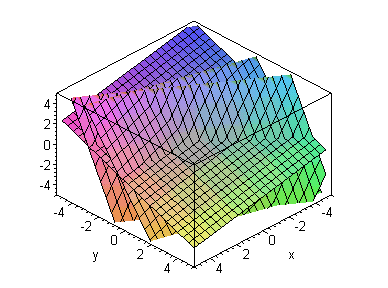Consistent solutions to three planes

Now let’s add to our system from the last blog and make a system of three equations; {2x + 3y – 3z = 14 , –3x + y + 10z = -32, x + 7y + 4z = -4}. If we try to solve this system by the inverse method we get the dreaded “Singular Mat” error on the calculator and all we can determine is that there is NOT a single unique solution. If we use the RREF we get something that should remind us of our previous example, and for good reason. Because I chose the third line to be a linear combination of the first two, the three planes all share a common line of intersection, the line (x, y, z) = (10, -2, 0) + t [3, -1, 1] as shown by the reduced form: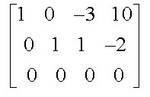The third row gives us an obviously true statement (0x + 0y + 0z = 0) that reflects that the third equation, in essence, added no new information to the system. This “row of zeros” at the bottom is the key to the student that the system had three equations but the intersection would be completely determined by any two of them.

When three planes intersect in space, there are six basic configurations (They sometimes miss the sixth possible case that all three equations are for the same plane) . I ask students to visualize and describe the different situations that can exist prior to discussion of the method of finding the solutions. In most years they come up with all five configurations and names for them. Here is a list of the five/six as my students tend to describe them.

The two consistent systems are three planes intersecting in a single point, and three planes intersecting in a single line (the book binding model – the planes are pages in a book and the common line is the binding).

The three configurations with no common points of intersection are three parallel planes, two parallel planes cut by a transverse plane (the H configuration) forming two parallel lines, and three planes intersecting in mutual pairs to form three parallel lines (the delta D configuration). The first and the second are often easy to recognize from the equations since the parallels are usually recognizable, but the third is difficult to identify without reduction.

Three configurations w/o a common solution
If we look at the three inconsistent systems possible with three planes, the more obvious cases may lead us to more understanding of the less obvious cases. First I want to look at three parallel planes, x + 2y + 3z = 1, x+ 2y + 3z = 5, and 2x + 4y + 6z = 10.
The reduced RREF augmented matrix looks like this:The first row looks like it is directly related to the coefficients of x, y, and z in each equation, but the 0 in the constant term may seem perplexing. I think of this information as a direction vector [1,2,3] that is perpendicular to the three parallel planes. The bottom line of zeros reminds us that the last equation added no new information about the solution set. But what are we to make of the equation for the second row, 0x + 0y + 0z = 1. I tell students that this is the signal that there is NO common point of intersection. In essence, we have an intersection when 0=1, and that is never true. If the three equations had all represented the same plane, then the original line would have contained the constant term as well [1, 2, 3, 1], and is in essence, just a version of the original plane.

Now we examine a system with two of the same parallels and a third plane not parallel to them, the H configuration, { x + 2y + 3z = 1, x+ 2y + 3z = 5, 2x – y + z = 1}. The reduced matrix looks like .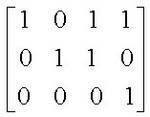How can we interpret this. The bottom row is our old insolvable friend 0x +0y + 0z = 1, reminding us that there can be no common point on the three planes (since two of them are parallel). The upper two rows look like the rows when we had a line of intersection except that both constant terms equal zero. Does this tell us anything about the two lines of intersection formed by the plane cutting through the two parallel planes. Perhaps we can find out more if we find the two intersections one at a time. If we reduce the two equations x + 2y + 3z = 3, 2x – y + z = 1, our reduced matrix looks like this,This can be read as the system of equations x+z=1 and y+z=1... so setting z = 0 first we get a point (1,1,0) on the intersection, and using z=1 we get (0, 0, 1)

. This gives a line (x, y, z) = (1, 1, 0) + t 1, 1, -1] . If we do the intersection with the second parallel plane we get (x, y, z) = (1.4, 1.8, 0) + t [1, 1, -1]. It seems that our original reduced system was flashing the direction vector part of the two parallel lines created by the three planes in the third column.

For our final case, we pick the inconsistent situation which is most difficult to distinguish from the consistent cases, the delta D configuration in which three planes intersect in mutual pairs to form three parallel lines. An example is the three planes defined by { x + y + 7z = 5, x – y – z = 3, and 2x – y + 2z = 4} .

The reduced form looks like this,and we are now becoming accustom to the 0001 on the bottom reminding us there is no common point for the three planes. And a quick inspection of the three planes assures us that no two of them are parallel, so the only possible case is the “delta formation” and three parallel lines of mutual intersection. What are we to make of the two upper rows. Our experience now leads us to think that [3, 4,-1] will be the direction vector for all three lines. We can verify our supposition by taking the vectors in pairs and reducing the two-equation systems to find the actual line of intersection, but as is the fashion in mathematical writing, I leave that final task as an exercise for the reader.

## Monday, 9 March 2009

### Planes in Space- part One

Take your basic bright kid in alg II or pre-calc, or often in calculus, and ask, "What is the intersection of two lines?"... They say, "A point."... good answer.

"Can you write the equation of a line?" Again they are on target.
"If I give you the equation of two lines on the plane; can you find their point of intersection?" The good ones can, and know they can.

Now ask the same bright kids, "What is the intersection of two planes in space?". They answer correctly again, "A line."
So far everything is great, but now we ask them to write the equation of a plane.... uhhh... gee..... and at this point, when asked about one of the fundamental structures of plane geometry, their analytic geometry skills are exhausted. Still, a very few may actually be able to produce x+y+z=1 or some other for the equation of a plane. Now we ask about the intersection of two planes, and almost none of them can do it. The scary part, is that very (very) few of the teachers of alg II and above that I have questioned about this could provide an answer either .
We begin by recalling that an equation in three variables, such as 2x+3y+z=6 can represent a plane in space. When students had three such equations that intersected in a unique point, they found the solution by one of several methods. Most students learn to solve such equations by the methods called elimination and substitution at the very least. Others may have also been introduced to Cramer’s rule for solving systems with determinants and perhaps two methods using matrices.

The most commonly taught matrix method is to write a matrix equation and then solve it using the inverse matrix method. A second, and as we will point out, more efficient and general method is the Gauss-Jordan reduced row-echelon form (RREF) of an augmented matrix. We give an example of both below to clarify the terminoligy.

We begin with three planes determined by the equations {x + y – 2z = 9; 2x – 3y + z = -2; and x + 3y + z = 2} This same system of equations can be expressed as the matrix equation.Notice that the left matrix is made up of the coefficients of the three variable terms in each equation, and the right matrix contains the constant terms. We can find the intersection by taking the inverse of the left matrix and multiplying on the left of both sides of the equation. The simplified result givesThis seems to be the most commonly taught method, and the one that students and teachers seem to prefer, and yet it has two major disadvantages. The first disadvantage is that it tells you little or nothing about systems which have a solution, but not a single unique solution. In fact it most students (or teachers?) can not distinguish between the cases (and there are several different ones) with no solutions frorm the ones with an infinite number of solutons. This same defect applies to attempts to use Cramers Rule. The second problem is that the inverse method is more computationally complex, that is, it takes more operations for the solution than the alternative RREF method, and the difference grows as problems reach higher orders of magnitude. For the problems that are generally assigned at the high school level, the difference in computability presents no real problem, but the difference in the range of applicable questions can be very significant in a students understanding of general systems of three equations.

In contrast with the Inverse method that will only work if the three planes intersect in a single point, the RREF form will allow us to work with systems which do not even have the same number of equations as unknowns. This is the type of situation created when we try to find the line of intersection of two planes.

RREF for two planes

We will use the equations 2x + 3y – 3z = 14 and –3x + y + 10z = -32. When we write an augmented matrix for the system of only two equations we get a 2x4 matrix, shown here:When we reduce this system, by matrices or otherwise, we getwhich is a matrix expression of x - 3z = 10 and y + z = -2.

We notice that both equations contain a z variable, it might occur to us to ask, “What happens if we substitute different values in for z?”. For example, if we try z=0 we note that from the first equation we get x=10 and from the second we get y=-2. What does this tell us about the point (10, -2, 0). If we check it against the two original equations we notice that the point makes both equations true, 2(10) + 3(-2) – 3(0) = 14 and –3(10) + (-2) + 10(0) = -32. So the point (10, -2, 0) is on both planes and therefore must lie on the line that is their intersection.

Can we find more points? What happens if we try z=1 or z=2 or other values. Using z=1 we get x – 3(1) = 10 which simplifies to x=13 ; and y +(1) = -2 which simplifies to y=-3. Checking the point (13, -3, 1) we see that it also makes both equations true, and so it must also be on the line of intersection.

Writing the parametric equation of a line in three space

So now we have two points on the line of intersection; (10,-2, 0) and (13, -3, 1). How can we describe the line? One way is to write a formula for all the points so that someone could find as many values of (x,y,z) as they wish. We do this with parametric equations. A parametric equation is an equation that explains the values of one set of variables (in this case x, y, and z) in terms of another “parameter” which we will call t. We will define the line in terms of one point, and instructions to get from one point to another; sort of a three-space equivalent of slope.

When we worked with slope in a plane we had to find the change in x and the change in y, but now in three space we need a change in z also. If we look at our two known points, we can see that from the first we found, (10,-2, 0), to the second, (13, -3, 1), the x-value increases 3, the y-value decreases 1, and the z value increased 1. Let’s record those as a ordered triple, but to keep it separate from our points we will use brackets to enclose the changes; like this [3, -1, 1] .

We can even use this to find more points. If we add [3, -1, 1] to the last point we found (13, -3, 1) we get (16, -4, 2); and we know it is on both planes because it makes both the original equations true (Remember? They were 2x + 3y – 3z = 14 and –3x + y + 10z = -32) . Of course we do not have to add integer multiples of t, and if we allow t to be ANY real value, then we can write a general expression for all the points on the line of intersection in the form (x, y, z) = (10, -2, 0) + t [3, -1, 1] . [some texts will write this as a vector form (x, y, z) = (10+3t, -2 – t, t ) ].
And we have written the equation of the line of intersection. One of the nice things about the matrix approach is that it allows us to focus on interpreting the results... do three planes intersect in a line, in two parallel lines, in three lines all heading in the same direction? I will try to talk about these issues next, and then a little more about the equations of planes in space.

## Saturday, 7 March 2009

### Take a Look at a Trillion Dollars

In this period of huge financial losses and bailouts, people throw numbers like a trillion dollars around rather casually. I am reminded of the quote by someone ?? who said, "A billion dollars here, a billion dollars there, and pretty soon that adds up to real money."
Well, I came across a nice illustration of what a trillion dollars would look like on a page over at Page Tutor and I copied a couple of the pictures to give you an idea:

"We'll start with a $100 dollar bill. Currently the largest U.S. denomination in general circulation. Most everyone has seen them, slighty fewer have owned them. Guaranteed to make friends wherever they go. A packet of one hundred$100 bills is less than 1/2" thick and contains $10,000. Fits in your pocket easily and is more than enough for week or two of shamefully decadent fun. " Here is what$1 million dollars would look like: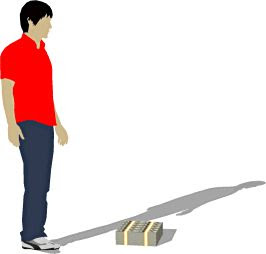"While a measly $1 million looked a little unimpressive,$100 million is a little more respectable. It fits neatly on a standard pallet..."With 100 of those pallets, you would have \$1 billion dollars... now we are talking real money.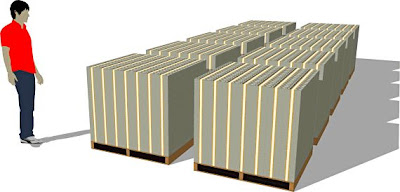And then????
"Next we'll look at ONE TRILLION dollars. This is that number we've been hearing about so much. What is a trillion dollars? Well, it's a million million. It's a thousand billion. It's a one followed by 12 zeros. And notice those pallets are double stacked.)"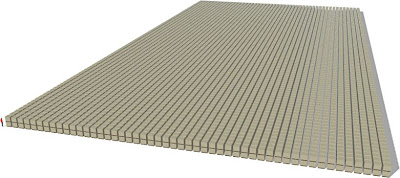Look carefully, there in the bottom left corner is our six-foot tall human, dwarfed by the staggering ocean of money... That's fifty pallets wide, 100 pallets deep, and two pallets high.... 10,000 pallets of 100 milliion each..... so next time someone talks about a trillion dollar bailout...close your eyes and imagine this warehouse full of money.
Now where did I park my fork lift?

## Thursday, 5 March 2009

### I Think I KenKen, I Think I KenKen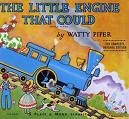I made the mistake of swinging by Dave Marain's MathNotations blog on a slow day... and there was that link to KENKEN.. Ok, I'm not really a big game bug.. I actually find Sudoku boring.. and those logic puzzles that match Ms. Jones as the Butcher married to Tom the Builder???? No way.. but for some reason I flicked one up and played around ... and then, what the heck, I wasn't in a hurry, so I did a bigger one... and then a harder one... then the really tough one and it took a while even giving in to get clues....
So you have been warned... the game is really addictive...but if you STILL want to try.. I have a permanent link on the sidebar just below my profile picture....and here is one if you just can't wait...... have a ball. They upgrade it each week with a few more games, although I thing you could play some of the hard ones over and over trying not to get hints...
Here are the rules, although they are right on the game...Click to enlarge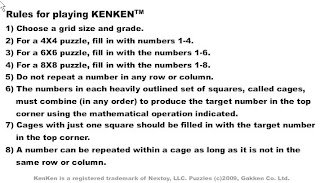## Wednesday, 4 March 2009

### Does Your Doctor Know Statistics

Conditional probability is hard,; doctors, it seems, don't understand the implications of it with tests they do on a regular basis. In the new book, The Numbers Game, (with a long sub-title) by Michael Blastland and Andrew Dilnot,(see below, and order here and I will donate the profit to charity)... they report that only two of twenty-four physicians could correctly work out the probability that a woman has breast cancer given that she tests positive on a mammagram.

Here are the details as they give them in the book, see if you can figure it out. We know that about 0.8% or .008 of all women in the forty-fifty age group have the condition (I haven't checked that figure, that is eight out of every thousand women, will follow up... I promise). The test is 90% accurate at spotting a person who has the disease... if you have it, 9 times out of ten they will catch it. The false positive rate is only 7%. If you don't have it, there is a 93% chance they will give you a clear bill of health. So you go to the doctor and he gives you that long face and says, "Sorry, you tested positive for breast cancer." Now the million dollar question.... .what is the probability that you really have it? Out of all the women in the forty-fifty age group who get that mind numbing statement, what percent REALLY has cancer?

If you are in my stats class, or ever were, you should know how to solve that, and it seems even a little shocking that some (they actually said "Most were not only wrong, but hopelessly wrong."

My way of addressing these kinds of problems is to make a table, and figure out wher all the labels go. The problem assumes that only 8 out of a thousand women really have it, so if we assume a million tests, 8,000 women really have cancer... 992,000 do not. We put this in the table.Now let's analyze what the test say.... of the 8,000 poeple who DO have cancer, the test will catch 90% or 7,200 of these people (the real victims are the 800 who walk away thinking they are clear and the cancer goes untreated). So we put these numbers in the correct squares of the table.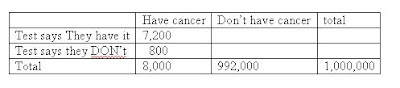Now we want to look at the much larger group who do not have cancer; even though the test is 93% accurate in identifying those who are free from cancer, it will falsly tell 7% of the group, or 69.440 of these people that they tested positive for the cancer. We include this information in the table here.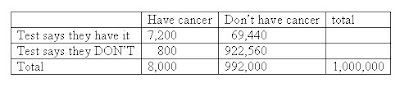Now we want to look at just the people who got a notice that they tested positive, and we see that there are 76,640 of them; but most of them, 69,440 of them, were false positives. Because such a large group do not have the disease, the number of false positives has swamped the true positives, and less than ten percent of these women really have cancer.I hope that if these nubmers are anywhere near the true probability, if someone tests positive, the doctor will at least say, "Hey, most of these are false positives..but just to be sure, let's look at a second test..because the probability of another false positive is only 7% (it hasn't changed just because you already had one false positive).. so most of these people will get a clean bill of health on the second trial, but amazingly, there will still be more women who receive a second false positive (46,860) than there were actual sufferers of the disease; over six times as many... maybe there are a few questions you want to ask your G.P. ; like , "When was the last time you took a statistics course?".

## Tuesday, 3 March 2009

### Tiny Circles

Just reviewing a note about the wonderful property of pinholes. If you are in a well lighted room, look at a sign or picture far across the room. If your eyes are old like mine, it may not be very clear. If you have great eyes, pick out a small object that is difficult to see clearly. Now punch a small hole in a 3x5 card or piece of paper with a thumbtack or such. Hold it up in front of the eye and look at the object through the hole. If you move it back and forth a little, you should notice the object is in much sharper focus... WHY???

As light strikes the eye it has to be brought to bear on a single point on the retina to foucs sharply. By looking though a tiny hole, the light is all falling on a very small spot, and thus is much sharper. It does reduce the total light, so the object has to be pretty bright.

You can do the same thing by squeezing the thumb and finger together (like you are signing OK) on both hands and then push the two thumbs and fingers together. They form a little diamond shaped hole in the center, and if you peer through this, it will have the same effect. Press a little harder to make the hole smaller and the image sharper. I use this sometimes in the underground to be able to read the tube maps on the opposite wall when they aren't clear.... and it works..

I just learned that "it was the 10th-century Arab physicist, astronomer and mathematician, Ibn al-Haytham (Alhazen), who published the principle of the pinhole camera in the Book of Optics in 1021 AD. He also invented the first pinhole camera after noticing the way light was streaming through a hole in a window shutter. He improved on the camera after realizing that the smaller the pinhole, the sharper the image. He went on to designed the first camera obscura (Latin for something like Shadow room).

## Sunday, 1 March 2009

### We Just Don't Talk That Way Anymore

I love reading old journals and am often struck by the precision and beauty of language in old math and science journals.

Many brighter than I have commented on the seemingly inevitable reduction in rigor as schools require all students to take more advanced math. I think the same thing has happened across the board to language as everyone is expected to complete a high school education. I recently read a note that had two quotes, both saying essentially the same thing. The first is from Leonhard Euler and dates around the American revolution; the second is from George Box and dated around 1987Empirical Model-Building and Response Surfaces. Both are incredibly literate and knowledgable people, and yet..

"Although to penetrate into the intimate mysteries of nature and thence
to learn the true causes of phenomena is not allowed to us, nevertheless
it can happen that a certain fictive hypothesis may suffice for
explaining many phenomena"

All models are wrong... some models are useful